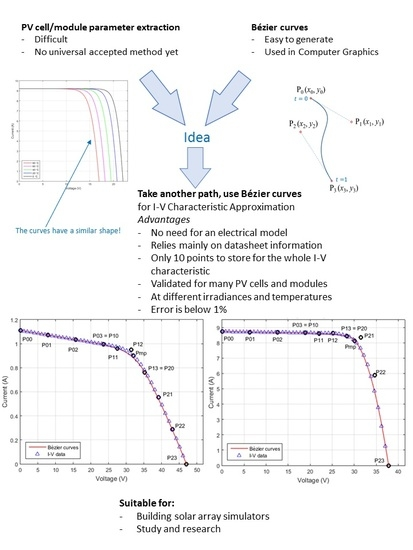Previous Article in Journal
Deep Neural Networks for Document Processing of Music Score Images
Article

Photovoltaic Cell and Module I-V Characteristic Approximation Using Bézier Curves

Applied Electronics Department, Politehnica University Timisoara, Timisoara 300006, Romania
*
Author to whom correspondence should be addressed.
Appl. Sci. 2018, 8(5), 655; https://doi.org/10.3390/app8050655
Received: 23 March 2018 / Revised: 30 March 2018 / Accepted: 12 April 2018 / Published: 24 April 2018
The aim of this work was to introduce new ways to model the I-V characteristic of a photovoltaic (PV) cell or PV module using straight lines and Bézier curves. This is a complete novel approach, Bézier curves being previously used mainly for computer graphics. The I-V characteristic is divided into three sections, modeled with lines and a quadratic Bézier curve in the first case and with three cubic Bézier curves in the second case. The result proves to be accurate and relies on the fundamental points usually present in the PV cell datasheets: $V o c$ (the open circuit voltage), $I s c$ (the short circuit current), $V m p$ (the maximum power corresponding voltage) and $I m p$ (the maximum power corresponding current), and the parasitic resistances $R s h 0$ (shunt resistance at $I s c$ ) and $R s 0$ (series resistance at $V o c$ ). The proposed algorithm completely defines all the implied control points and the error is analyzed. The temperature and irradiance influence is also analyzed. The model is also compared using the least squares fitting method. The final validation shows how to use Bézier cubic curves to accurately represent the I-V curves of an extensive range of PV cells and arrays. View Full-Text
Show FiguresGraphical abstract

MDPI and ACS Style

Szabo, R.; Gontean, A. Photovoltaic Cell and Module I-V Characteristic Approximation Using Bézier Curves. Appl. Sci. 2018, 8, 655. https://doi.org/10.3390/app8050655

AMA Style

Szabo R, Gontean A. Photovoltaic Cell and Module I-V Characteristic Approximation Using Bézier Curves. Applied Sciences. 2018; 8(5):655. https://doi.org/10.3390/app8050655

Chicago/Turabian Style

Szabo, Roland, and Aurel Gontean. 2018. "Photovoltaic Cell and Module I-V Characteristic Approximation Using Bézier Curves" Applied Sciences 8, no. 5: 655. https://doi.org/10.3390/app8050655

Find Other Styles
Note that from the first issue of 2016, MDPI journals use article numbers instead of page numbers. See further details here.

1